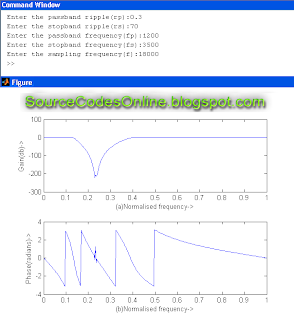# MATLAB Program for IIR(Infinite Impulse Response) Band Stop Filter using the Window function | IT1254 - DSP and Communications Systems Lab

AIM:
To write a program for IIR band stop filter using the window function using MATLAB in IT1254 - DSP and Communications Systems Lab.

ALGORITHM:
Step 1: Start
Step 2: Read the pass band ripple
Step 3: Read the stop band ripple
Step 4: Read the pass band frequency
Step 5: Read the stop band frequency
Step 6: Read the sampling band frequency
Step 7: Read the beta value
Step 8: Perform band stop filter calculations
Step 9: Plot the output sequence
Step 10: Stop

PROGRAM:
clc;
close all;
clear all;
format long;
rp=input('Enter the passband ripple(rp):');
rs=input('Enter the stopband
ripple(rs):');
fp=input('Enter the passband frequency(fp):');
fs=input('Enter the stopband frequency(fs):');
f=input('Enter the sampling frequency(f):');
wp=2*fp/f;
ws=2*fs/f;
[n]=buttord(wp,ws,rp,rs);
wn=[wp,ws];
[b,a]=butter(n,wn,'stop');
w=0:0.01:pi;
[h,o]=freqz(b,a,w);
m=20*log10(abs(h));
an=angle(h);
subplot(2,1,1);
plot(o/pi,m);
ylabel('Gain(db)->');
xlabel('(a)Normalised frequency->');
subplot(2,1,2);
plot(o/pi,an);Click to view full size image!